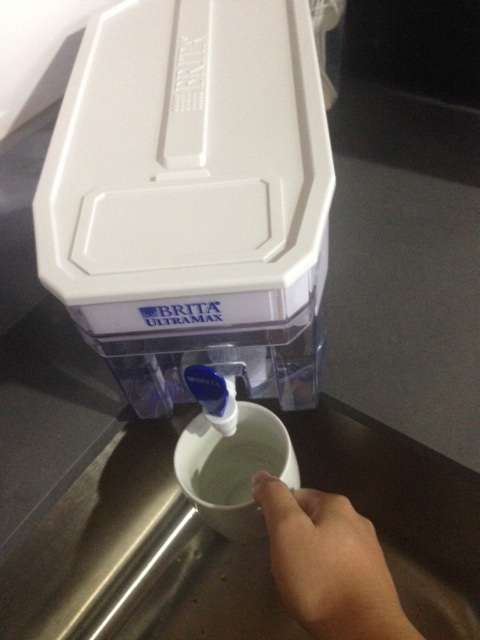# A conversation at the Brilliant water cooler

Logic Level 3Suyeon is thinking of 2 (not necessarily distinct) positive integers, $x$ and $y$, each of which is greater than 1. She tells Calvin the product $P = xy$ and Aaron the sum $S = x + y.$ The equally intelligent Calvin and Aaron then engage in a short discussion as follows:

• Calvin: "I cannot determine $S$ at this point."

• Aaron: "All right then, here's a hint; $S$ does not exceed $20$, and if that's all you need to know to uniquely determine $S$ then I will know what $P$ is."

• Calvin: "O.k., based solely on the fact that $S$ does not exceed $20$ I am now able to uniquely determine $S$."

Find $S + P$.

###### This question was inspired by the long-suffering Calvin Lin (inside joke).
×

Problem Loading...

Note Loading...

Set Loading...